### Home > CAAC > Chapter 8 > Lesson 8.2.5 > Problem8-89

8-89.
1. Calculate the expressions below. Then compare your answers from (a) and (b) to those in problem 8-88. What do you notice? Homework Help ✎

1.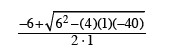2.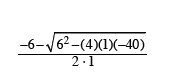3.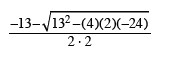4.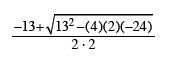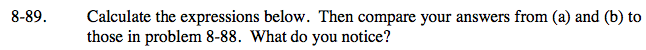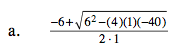$\frac{-6+\sqrt{36+160}}{2}$

$\frac{-6+\sqrt{196}}{2}$

$\frac{-6+14}{2}$

$\frac{8}{2}$

4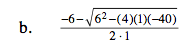Follow the steps in part (a).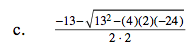Follow the steps in part (a).

−8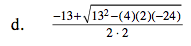Follow the steps in part (a).

The answers you get for parts (a) through (d) will match those found in problem 8-88.